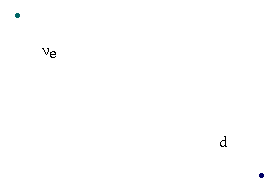# The Weak Force

## It's Weak!

The most dramatic difference between the weak force and the electromagnetic force is in its strength. As its name would imply, the weak force is much weaker than electromagnetism or the strong force. However, what we mean by the strength of a force is not always the same on a microscopic level as it is on a macroscopic level. In the macroscopic world, we think of the strength of a force in terms of so many pounds (or Newtons or dynes, if you're metrically inclined) of force that one object exerts on another. But in the microcosmos, there's a different way of thinking of strength.

When two subatomic particles approach each other, they may or may not interact with each other at all. This depends on the distance at which the particles approach, the nature of the force between them, and random chance. A pion and proton, which interact via the (residual) strong force, are very likely to interact with each other, if they approach within range. An electron and a proton, which interact electromagnetically, are much less likely to. Hence we say that the strong force is much stronger than the electromagnetic force. This is not because the interaction between pion and proton produces a much larger effect (it doesn't), but because such interactions between pion and proton are much more likely than between electron and proton.

So when we say that the weak force is weak, it's because two particles that can only interact weakly with each other are, in fact, very unlikely to interact at all. How unlikely? Well, let's suppose for the moment that we build a really large aquarium. An aquarium 1000 light-years long. Now we fill this aquarium with water, and fire a neutrino into one end. A neutrino is a good particle for illustrating the weak force, because it's colorless and electrically neutral, so that the only force we need concern ourselves with is the weak force. (Gravity is a whole other kettle of fish.) Anyway, this neutrino, which can only interact with the other particles in the aquarium via the weak force, has a fifty-fifty chance of passing all the way through this aquarium without hitting anything!

I'll pause here a bit while you read that last paragraph again, and gape at the last sentence. It's a truly staggering stat.

The sun spews out neutrinos in huge numbers. This poses no danger to us, however, because of the fact the vast, vast, vast majority of them pass right through us with absolutely no effect whatsoever. One might well ask why the weak force is so much weaker than the others. Well, as with the other forces, the characteristics of the weak force spring from the characteristics of its mediating particles, the W± and the Z0.

## Mass and Range

The fact about the W's and Z that make the weak force most distinctive is that they are very heavy. The W's are about 80 times as massive as a proton, and the Z is even a bit heavier. You may recall that the mass of the mediating particle determines the range of the force. So, the huge mass of the W's and Z lead to a force that has a very short range. And in fact, it's this shortness of range that leads to the weakness of the weak force. Within this very short range, the weak force is actually no weaker than the electomagnetic force. It's just that the range is so short, that the chance of an interaction between two weakly interacting particles is very slim. Hence the aquarium stat above.

## Change of "Flavor"

The fact that the W's are electrically charged is also new. And this leads to a unique feature of the weak force. Look at the following interaction between a neutrino and a quark. (The quark is presumably a part of some larger particle such as a proton or neutron, but I'll keep the picture simple.)The thing to notice about this process is that you start out with one type of quark, one "flavor" in the whimsical vernacular of particle physicists, and you end up with another. That is, the original d-quark is changed to a u-quark. Similarly, the lepton in the process changes flavor from an electron neutrino to an electron.

This flavor change occurs in any process involving a W particle. You see, since the W has electric charge, this means that any particle that emits or absorbs a W (real or virtual) also emits the electric charge it carries. So you end up with a particle with a different charge, and so a different flavor. When the negatively charged d-quark absorbs the W, it gains its positive charge, and becomes the positive u-quark.

Because this property is unique to the weak force, this force is profoundly important in spite of its weakness. The nuclear fusion that takes place in the sun and is responsible for the heat it emits involves a series of processes in which four protons are converted into a helium nucleus: two protons and two neutrons. The conversion of protons into neutrons involves the conversion of a u-quark into a d-quark, and so the weak force is essential to solar fusion.

In addition, many of the particles I listed near the beginning of this website, such as the b-quark and the muon to name just a couple, are unstable. They decay into other particles. Like this muon here, which you've seen before:Notice that the first step in this process is that the muon emits a W particle and becomes a muon neutrino: a flavor-change, which can only occur by means of the weak force. Without the weak force, the muon, tau, s-quark, c-quark, b-quark, and t-quark might all be stable, the universe would be crawling with these things instead of almost devoid of them, and things would be much different.

Gravity
Previous page
Dave's Microcosmos